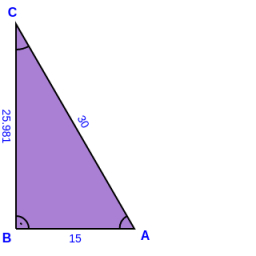# Perpendicular 40203

In a right triangle, one perpendicular is 5 cm longer than the other perpendicular. The diaphragm is 150 mm. Calculate the lengths of the hangings.

a =  12.8078 cm
b =  7.8078 cm

### Step-by-step explanation:Did you find an error or inaccuracy? Feel free to write us. Thank you!

Tips for related online calculators
Do you have a linear equation or system of equations and looking for its solution? Or do you have a quadratic equation?
The Pythagorean theorem is the base for the right triangle calculator.

#### Grade of the word problem:

We encourage you to watch this tutorial video on this math problem: